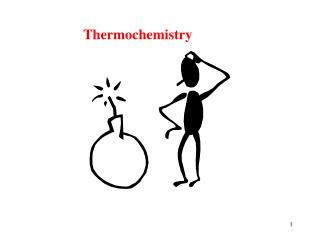DownloadDownload PresentationThermochemistry

# Thermochemistry

Download Presentation## Thermochemistry

- - - - - - - - - - - - - - - - - - - - - - - - - - - E N D - - - - - - - - - - - - - - - - - - - - - - - - - - -
##### Presentation Transcript

1. Thermochemistry

2. Thermochemistry Chemical reactions are accompanied by changes in energy.

3. Definitions: Energy: The ability to do work, w = f x d K.E. =1/2mv2 energy in motion What are some forms of kinetic energy? Mechanical, electrical, thermal, sound, etc (Motion) P.E. potential energy What are some forms of potential energy? Chemical Positional

4. Energy Units: Joule = J 1 J = kg ·m/s2 Since a J is very small we usually use kJ’s calorie = cal 1 cal = 4.184 J (exact by definition) 1 cal 4.184 J A conversion factor! 1 cal is the amount of heat necessary to heat 1 g of water 1oC (from 14.5 to 15.5oC)

5. Food Calories: 1 Cal (note capital for food) = 1000 cal = 1 kcal So... That donut with only 500 Calories is really.... 500,000 calories... (eat 2 to make it an even million).

6. 1st Law of Thermodynamics: Energy cannot be created nor destroyed. System  Surroundings System: whatever we are interested in. Surroundings: the rest of the universe

7. Internal Energy (E): The sum of all K.E. and P.E. of a system. Internal energy is too complex to be measured exactly, so we look at the change in internal energy: Esystem = Efinal - Einitial Sign of E: (+) means: system has gained energy (-) means: system has lost energy From the 1st Law of Thermodynamics: Esystem = -Esurroundings

8. Sign of E: (+) means: system has gained energy (-) means: system has lost energy 2H2(g) + O2(g)  2H2O(g) 2H2O(g)  2H2(g) + O2(g)

9. Sign of E: (+) means: system has gained energy (-) means: system has lost energy + energy (given off) 2H2(g) + O2(g)  2H2O(g) 2H2O(g)  2H2(g) + O2(g) + energy (added to system)

10. Heat and Work: energy exchange E = q + w Work done on (+) or by (-) the sys. Heat gained (+) or lost (-) by the sys. Internal energy of the sys. (both K.E. and P.E.)

11. Reactions are Energy Driven: Energy given off  Exothermic (-) sign “Exit” Energy absorbed  Endothermic (+) sign “Enter” Remember: 1st Law of Thermo.: Esystem = -Esurroundings So for every endothermic process there is an exothermic process.

12. Decide if each of the following are Endo or Exothermic processes: Ice melting? Water freezing? A candle burning? Dynamite exploding? A plant growing?

13. Misconception: Energy vs Temperature Both containers are at the same temperature, which one contains the most energy? Temperature is an intensive property (amount independent) Energy is an extensive property(amount dependent)

14. Enthalpy (H): heat content per amount Extensive property Enthalpy Change (H) In a reaction: H = HProducts - Hreactants H (+) endo H (-) exo

15. Enthalpy Change (H) In a reaction: H = HProducts - Hreactants H (+) endo H (-) exo Enthalpy is a state function. “Net Change” “Path indepedent” Both accomplished the same net result! A B

16. Problem: Given the reaction, which occurs in the presence of the catalyst MnO2): 2KClO3(s)  2KCl(s) + 3O2(g) H = -89.7 kJ a. What would be the value of H for the reverse RXN? +89.7 kJ

17. Problem: Given the reaction (which occurs in the presence of the catalyst MnO2): 2KClO3(s)  2KCl(s) + 3O2(g) H = -89.7 kJ The H value listed is really a conversion factor! -89.7 kJ -89.7 kJ -89.7 kJ 2 mol KClO3 2 mol KCl3 mol O2

18. Problem: Given the MnO2 catalyzed reaction: 2KClO3(s)  2KCl(s) + 3O2(g) H = -89.7 kJ -89.7 kJ -89.7 kJ -89.7 kJ 2 mol KClO3 2 mol KCl3 mol O2 Calculate the value of Hfor the decomposition 9 moles of KClO3 ? 2KClO3(s)  2KCl(s) + 3O2(s) H = -89.7 kJ 9 moles KClO3 -------------------------------------------------- = kJ -403.7

19. Problem: Given the MnO2 catalyzed reaction: 2KClO3(s)  2KCl(s) + 3O2(g) H = -89.7 kJ -89.7 kJ -89.7 kJ -89.7 kJ 2 mol KClO3 2 mol KCl3 mol O2 Calculate the value of H for the formation 25.0 grams of KCl . 2KClO3(s)  2KCl(s) + 3O2(s) H = -89.7 kJ 25.0 g KCl mol KCl -89.7 kJ -------------------------------------------------- = kJ -15.0 74.6 g KCl 2 mol KCl